# Using Ruler andSet Squares

1. Draw a line l and take a point outside the line.

2. Place any side of the set square forming the right angle along the line l.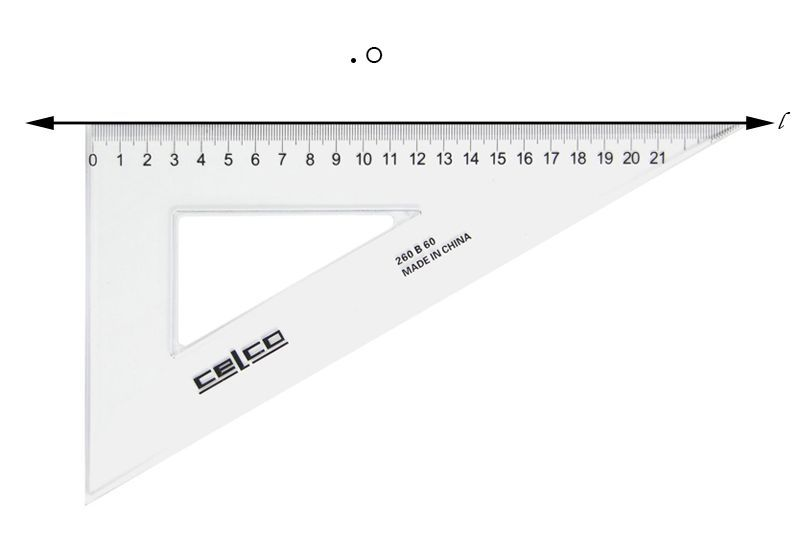3. Place the ruler along the other side of the set square forming the right angle as shown in the figure.

The ruler is to be kept fixed.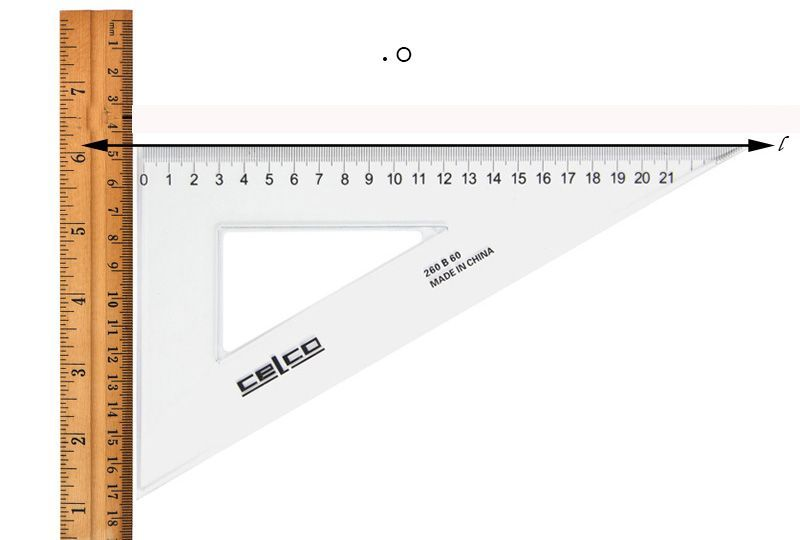4. Slide the set square along the ruler upwards such that the point O lines along the arm of the set square.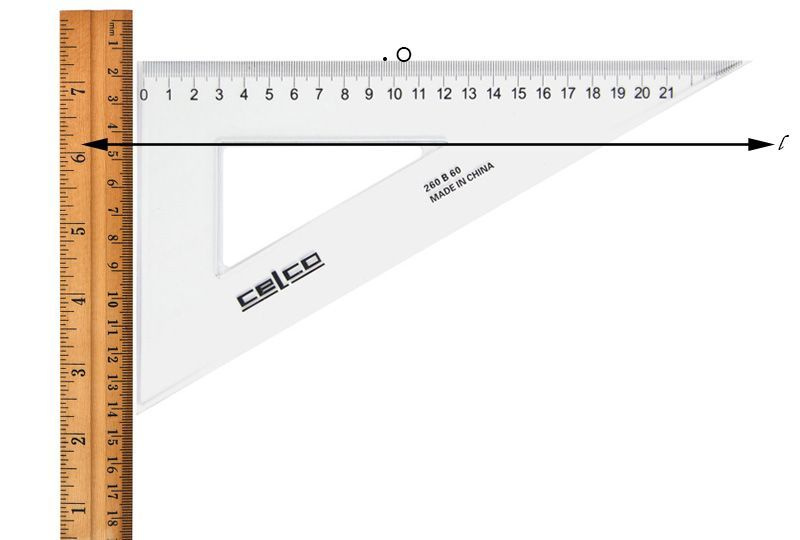5. Remove the ruler and draw a line along the set square. Name this line as m.

m is the required line parallel to l. m || l.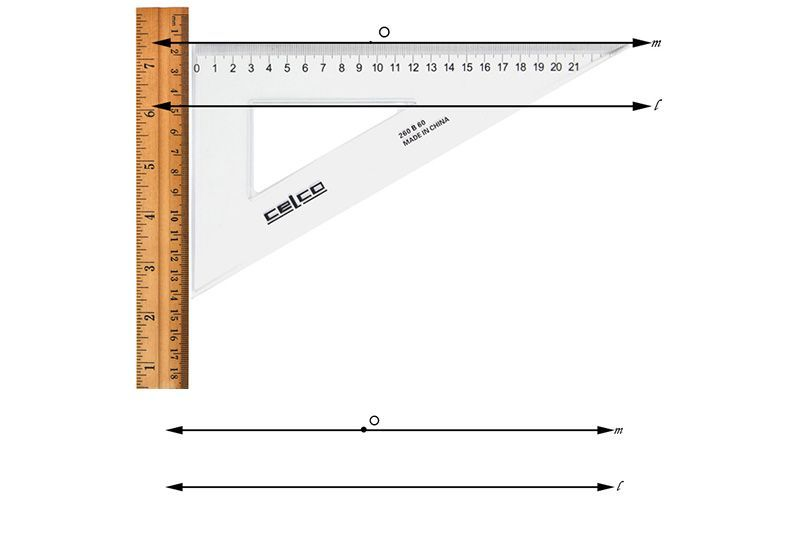# Using Ruler and Compass

1. Draw a line l and take a point A outside it.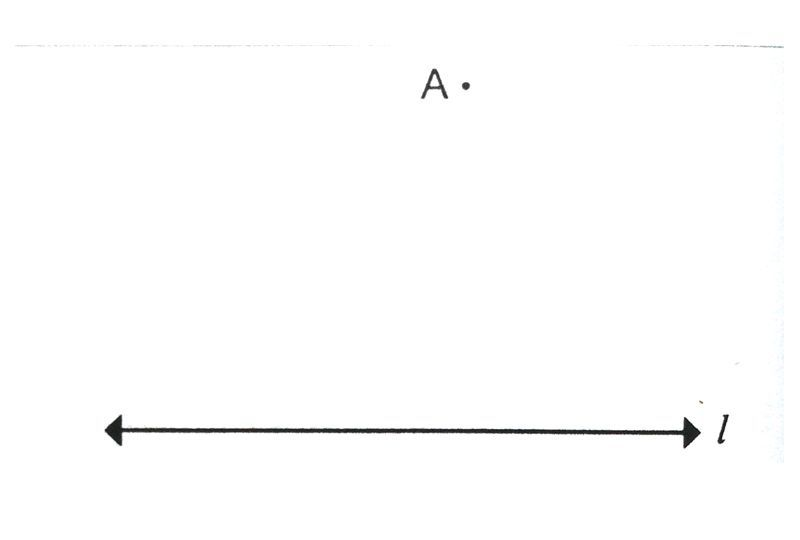2. Take any point B on the line and join A and B.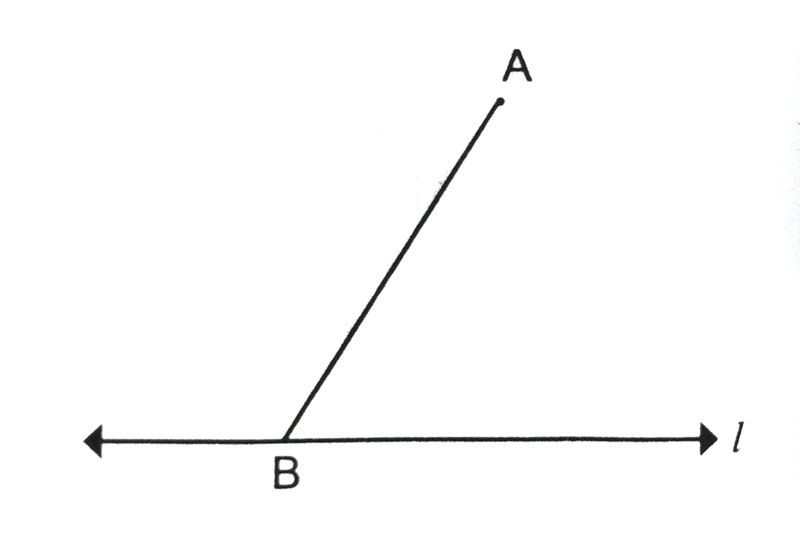3. With B as the centre and any convenient radius, draw an arc intersecting line l at P and AB at Q.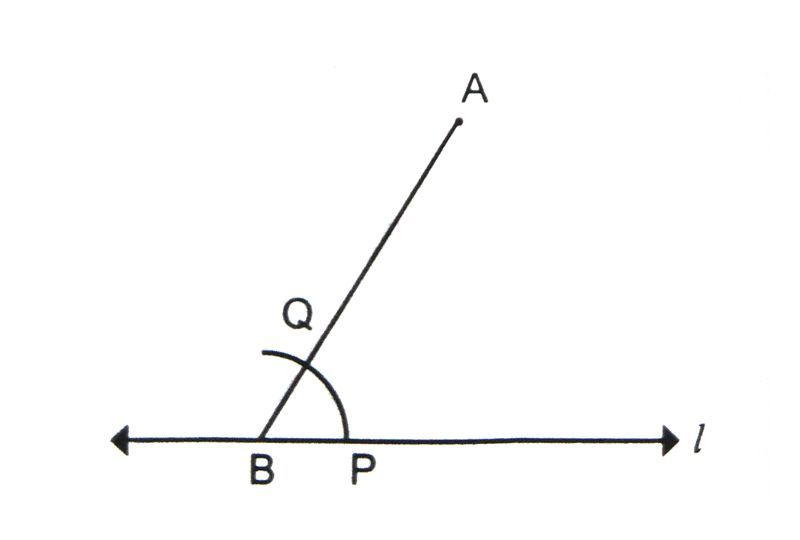4. With A as the centre and same radius, draw an arc to intersect AB at R.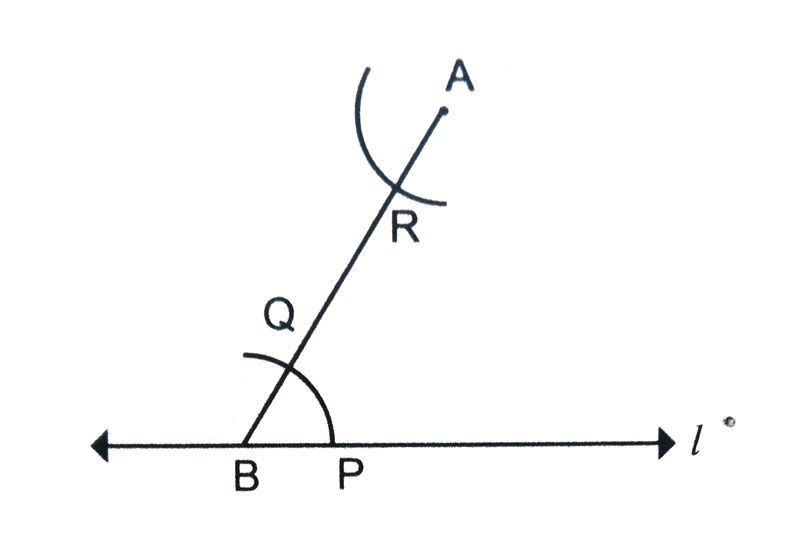5. With the compass measure the distance between the points P and Q.

6. With R as the centre, and radius equal to PQ (just measured). Draw an arc intersecting the previous arc at S.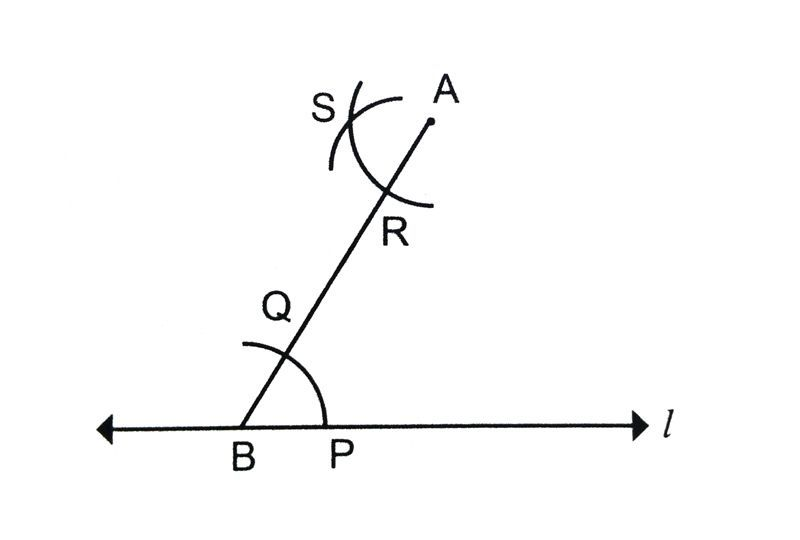7. Draw a line through A and S. m is the required line parallel to l passing through the point A. l || m.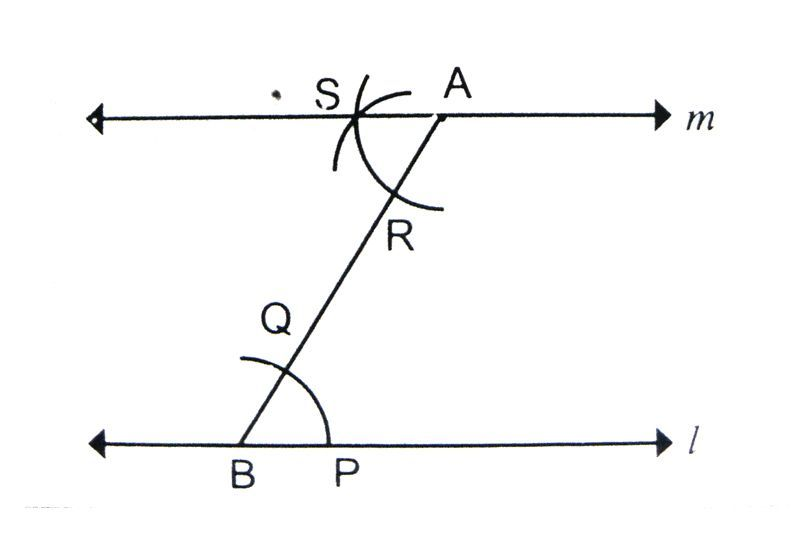# At a given distance

1. Draw a line l.

2. Construct a perpendicular to the given line.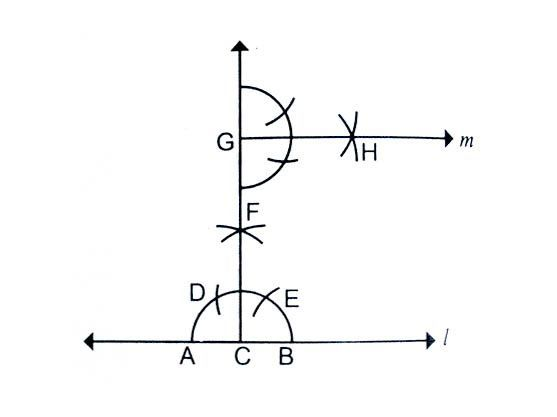3. Take a point at the given distance from the line on the perpendicular.

4. Construct a line parallel at that point using the previous methods.

# Access All Content

## More from Grade 7 Math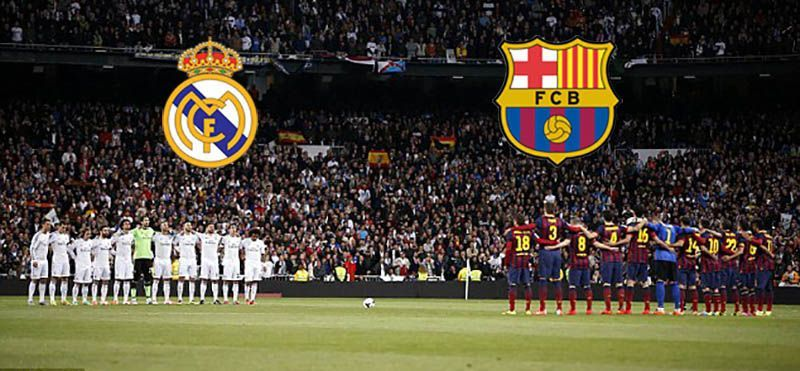# Sets

Sets are around us, everywhere. Learn how they are formed, named, and represented.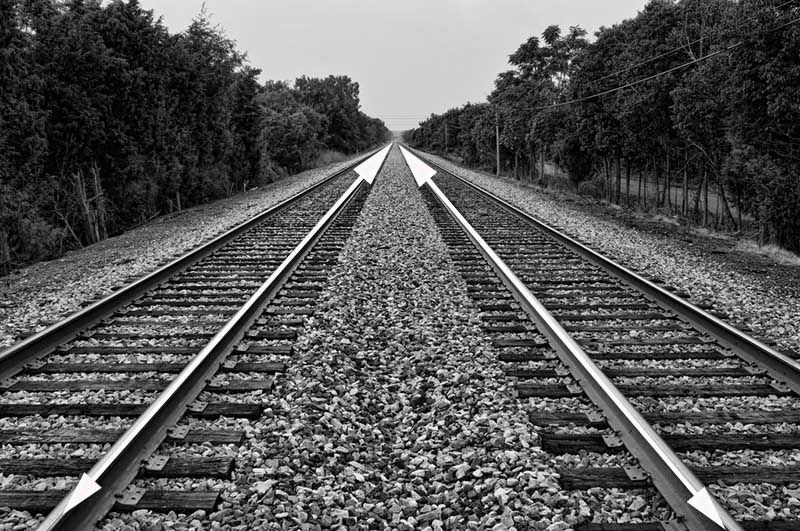# Types of Lines

All you need to know about lines, parallel, perpendicular and intersecting lines. Along with all the examples from the world around you.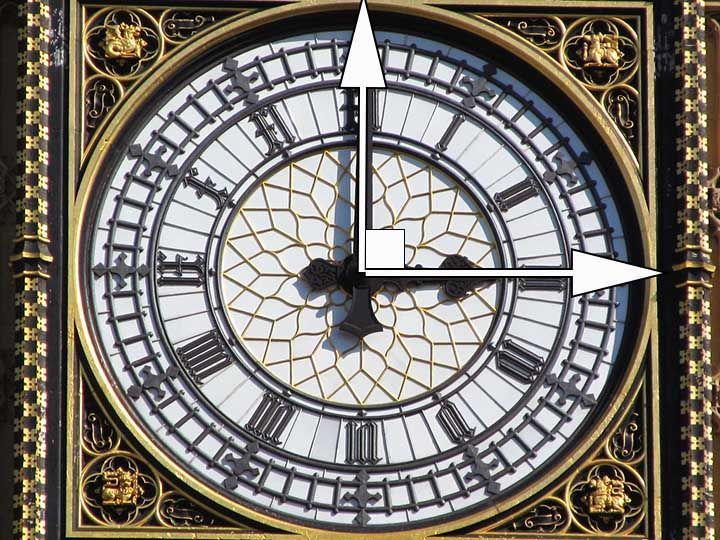# Types of Angles

Learn about the different types of angles acute, right, obtuse and more through exciting facts from the Wonders of the World.